•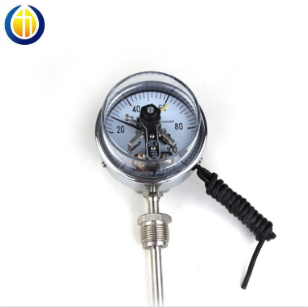双金属电接点温 `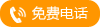` `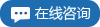`
•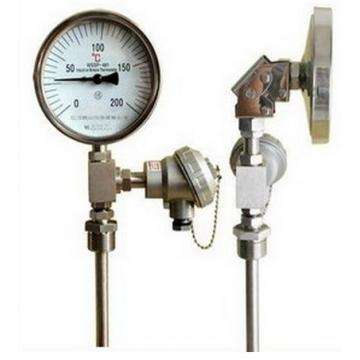双金属远传温度 `` ``
•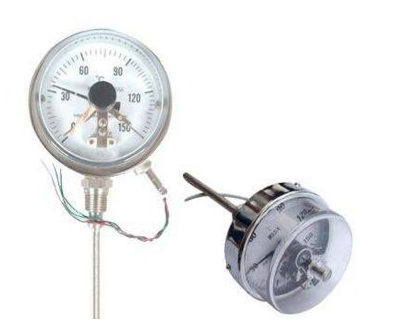防爆电接点双金 `` ``
•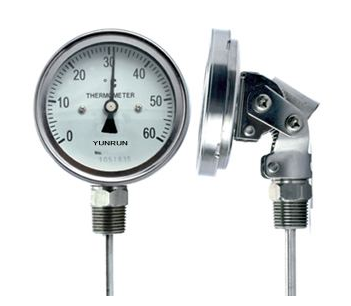万向型双金属温 `` ``
•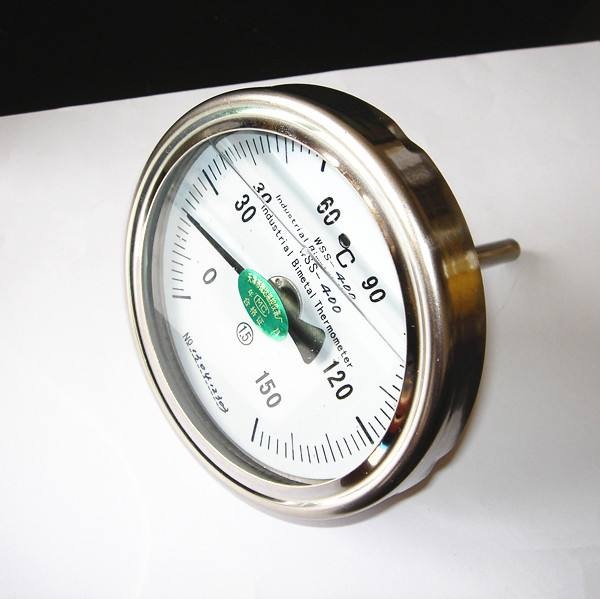耐震双金属温度 `` ``
•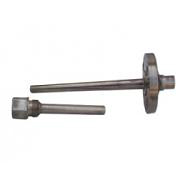温度仪表外保护 `` ``
•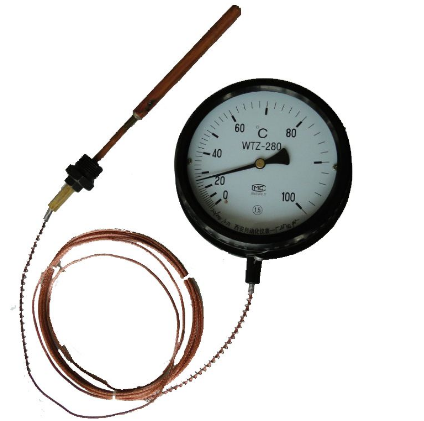压力式温度计 `` ``
•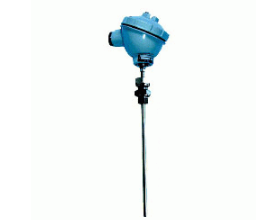装配式热电偶 `` ``
•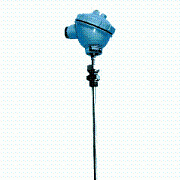铠装热电偶 `` ``
•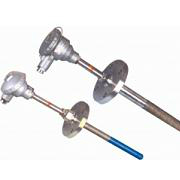耐磨热电偶耐磨 `` ``
•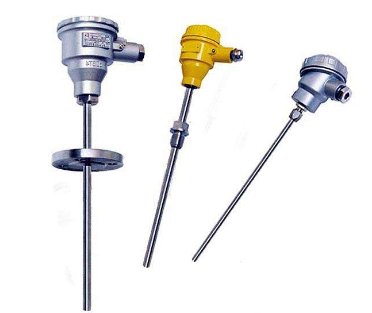多点热电偶 `` ``
•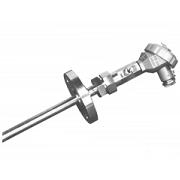吹气热电偶 `` ``
•装配式热电阻 `` ``
•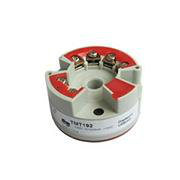智能模块式温度 `` ``
•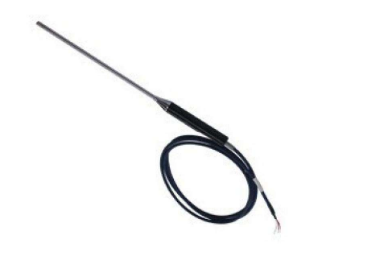J型热电偶 `` ``
•钨铼热电偶 `` ``
•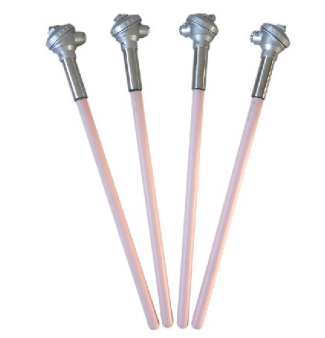S型热电偶 `` ``
•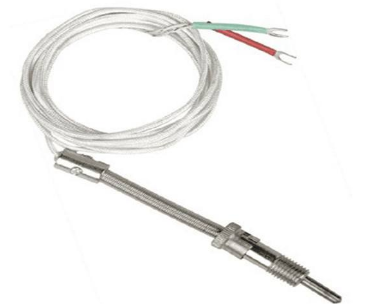压簧式热电偶 `` ``
•防爆热电偶 `` ``
•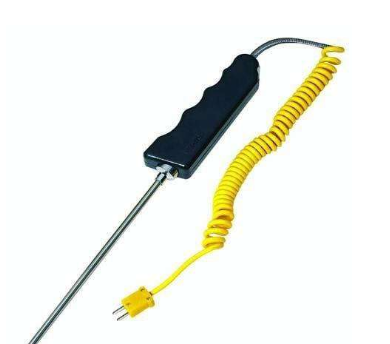手柄式铠装热电 `` ``